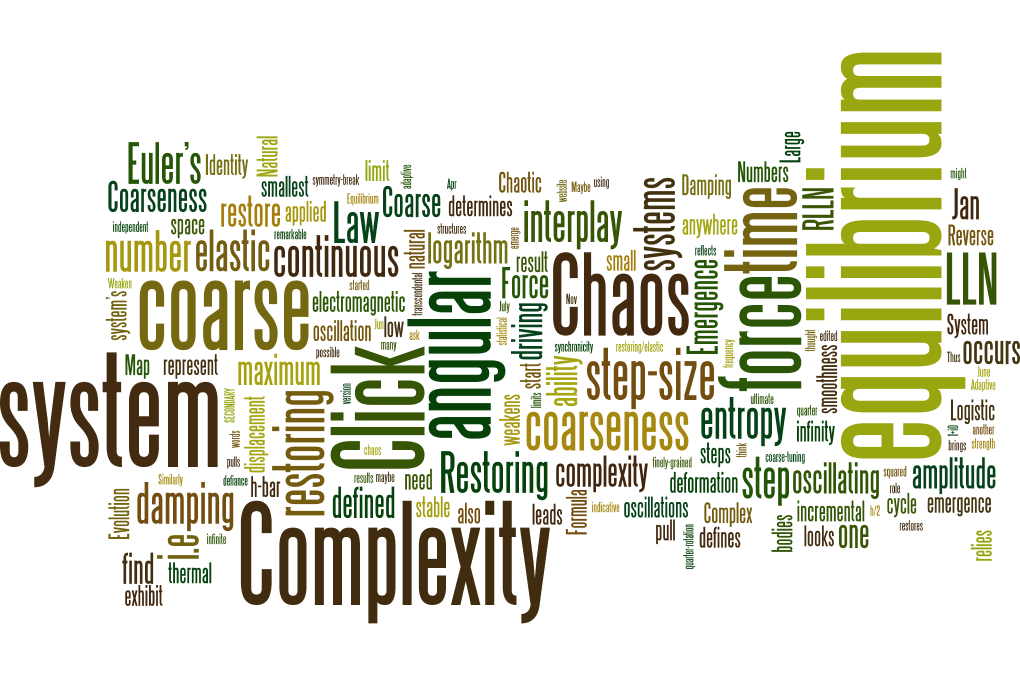# Timeline2004 – Complexity

• Complexity and Evolution seems to occur in defiance of the Second Law of Thermodynamics (SLOT), but the SLOT is actually really only a “probabilistic” law of nature and consequently it relies heavily on the strength of the “Law of Large Numbers” (LLN).
• Thus a stable thermal equilibrium relies on the LLN; and the stronger the influence of the LLN (i.e. the more independent elements in the system), the more stable will be the equilibrium.
• Adaptive Systems however exhibit inter-dependence and this leads to a Reverse of the Law of Large Numbers (RLLN))
• The RLLN (weakens the LLN and) pulls system ways from equilibrium (where ultimately the system can potentially symmetry-break).
• Complex System exhibit an interplay of LLN and RLLN, an interplay of entropy and reverse entropy.

[Click here for The Reverse Law and Emergent Dynamics]

Nov  2013 – Chaos

• Chaotic Behavior in Logistic Map is the result of the dual role of driving parameter (R). R not only determines the driving force, it also determines the “coarseness” of the incremental step from one iteration to the next.

[Click here for Coarse Synchronicity in the Logistic Map]

Jan 2014 – Coarseness

• The commonality between Chaos and Complexity is “Coarseness”. Coarseness restricts a system’s ability to find equilibrium.  Both chaos and complexity are forms of “coarse damping”.  Chaos is coarse damping in time (coarse synchronicity), complexity is coarse damping in structure (coarse entropy).

[Click here for What is Coarse Damping?]

Jan 2014 – Natural Damping & Coarse Equilibrium

• In the log map, for low values of R (i.e. low driving force, & finely-grained step-size) if you start the system from anywhere other than equilibrium, it will spontaneously restore to equilibrium.
• Similarly in a thermal systems of very many independent parts, if we start the system from anywhere other than equilibrium, or pull the system away from equilibrium, the system will restore to equilibrium.
• What sort of equilibrium these system restores to, is determined by the system’s ability to fine-tune to equilibrium

June 2014 – Natural Restoring Force

• Both Chaos and Complexity are Damped-Driven System, both are systems where coarseness in the system is resisting the pull of a “natural restoring force”. Restoring forces are indicative of both oscillating bodies and elastic materials.

July 2014 – Restoring Force in Chaos & Complexity

• Chaotic behavior emerges because of a coarse restoring force in oscillating bodies
• Complex structures emerge because of coarseness in the restoring/elastic force of entropy; in other words, complexity is a deformation of an elastic material.

Oct 2014 – Weaken Restoring Force

• Elasticity reflects a systems restoring force. Most elastic systems have a “elastic limit” where the restoring force fails.
• Both Chaos and Complexity occurs as a result of a weaken restoring force.
• Chaos occurs when the coarseness of the incremental step-size weakens the system ability to find or restore equilibrium.
• Complexity occurs when a adaptive system drives against the “entropic limit”, and cause some form of “elastic/entropic deformation”.

[Click here for The Emergence of Complexity]

[Click here for The Evolution of Complexity]

Dec 2014 – Emergence

• The emergence of both Chaos + Complexity results from the interplay of coarse-tuning and fine-tuning (i.e. the interplay of coarseness and smoothness).
• So if coarse damping brings about emergence, maybe everything is “coarse emergent” including space and time. Maybe space and time are also coarse?…

Jan 2015 – Time

• The ultimate in smoothness is continuous Space-Time. The transcendental number “e”, defined using limits to infinity, is usually used to represent continuous time.
• But “e” started life as the base of a logarithm, and the basic idea behind logarithm is very small steps applied a large number of times.
• “e” is the “natural logarithm” because the step-size is exact inverse of the number of steps, and defined effectively as an infinitely small step-size applied an infinite number of time. So “e” represent continuous time; but what if time where not continuous?

[Click here for What is e?]

[Click here for What is Euler’s Identity?]

Feb 2015 – Euler’s Formula

• If N did not go to infinity in the definition of “e”, then in Euler’s Formula we would find a quantum angular step.

[Click here for How Do You Like Them Apples?]

Mar 2015 – Is there a smallest angular step?

• What if a smallest angular step could be defined in terms of the maximum angular displacement (π) on the x axis.
• So if (-1)1 defines the maximum angular step, then (-1)x defines every other angular step, and so then all we need to do is ask the question “what value of “x” would we need in order to be the smaller possible step-size? Well let’s try h-bar…

Apr 2015 – “i”

• All rotations are oscillations, and all oscillations are cycles. (i) is a quarter-rotation, which means (i) is a quarter-cycle, and a quarter cycle of oscillation travels the same distance as the maximum displacement from equilibrium (i.e. the Amplitude of the oscillation).
• (i)2 looks very similar to amplitude squared (A)in the formula for simple harmonic motion…
• (-1) to the power of h-bar produces an angular step that looks remarkable like h/2

[Click here for What is Euler’s Secret Identity?]

Jun 2015 – “Cycle Amplitude”

• (-1)x is an “angular frequency” of electromagnetic oscillation
• (i) is the “cycle amplitude” of electromagnetic oscillation